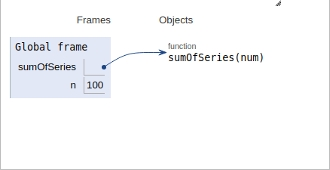# Python Program to find the sum of a Series 1/1! + 2/2! + 3/3! + 4/4! +窶ｦ窶ｦ.+ n/n!

In this article, we will learn about the solution and approach to solve the given problem statement.

Problem statement −Given an integer input n, we need to find the sum of a Series 1/1! + 2/2! + 3/3! + 4/4! +…….+ n/n!

Here we are implementing for loop, therefore, we get O(n) as the time complexity.

Here to reach the efficiency we calculate factorial within the same loop.

Here we frame a sumofseries function as described below −

## Example

Live Demo

def sumOfSeries(num):
res = 0
fact = 1
for i in range(1, num+1):
fact *= i
res = res + (i/ fact)
return res
n = 100
print("Sum: ", sumOfSeries(n))

## Output

Sum: 2.7182818284590455

All variables and functions are declared in global scope as shown in the figure below.## Conclusion

In this article, we learned about the approach to find whether it is possible to make a divisible by 3 numbers using all digits in an array.